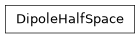# geoana.em.static.DipoleHalfSpace#class geoana.em.static.DipoleHalfSpace(rho, location_a=None, location_b=None, current=1.0)#

Class for a dipole in a halfspace.

The DipoleHalfSpace class is used to analytically compute the potentials, current densities and electric fields within a halfspace due to a dipole source. Surface is assumed to be at z=0.

Parameters
currentfloat

Electrical current in the point current (A). Default is 1A.

rhofloat

Resistivity in the point current ($$\Omega \cdot m$$).

location_aarray_like

Location of the A current source electrode (m). Default is (-1, 0, 0).

location_barray_like

Location of the B current sink electrode (m). Default is (1, 0, 0).

Attributes

 current Current in the dipole in Amps. location_a Location of the A current source electrode. location_b Location of the B current sink electrode. rho Resistivity in the dipole in $$\Omega \cdot m$$.

Methods

 current_density(xyz_m[, xyz_n]) Current density for a dipole source in a halfspace. electric_field(xyz_m[, xyz_n]) Electric field for a dipole source in a halfspace. potential(xyz_m[, xyz_n]) Electric potential for a dipole in a halfspace.